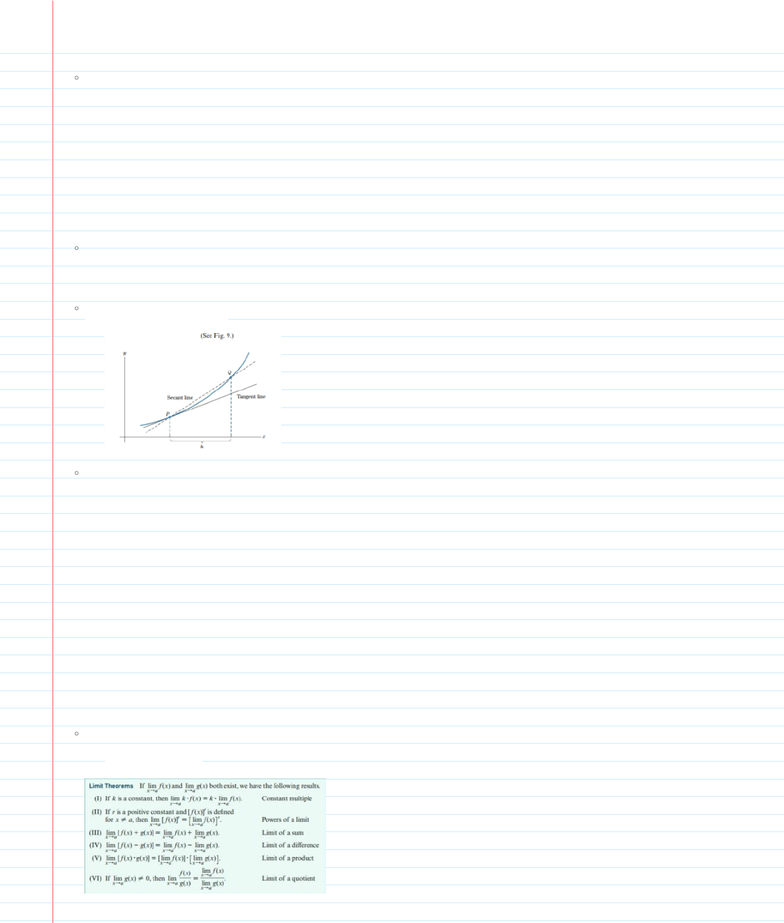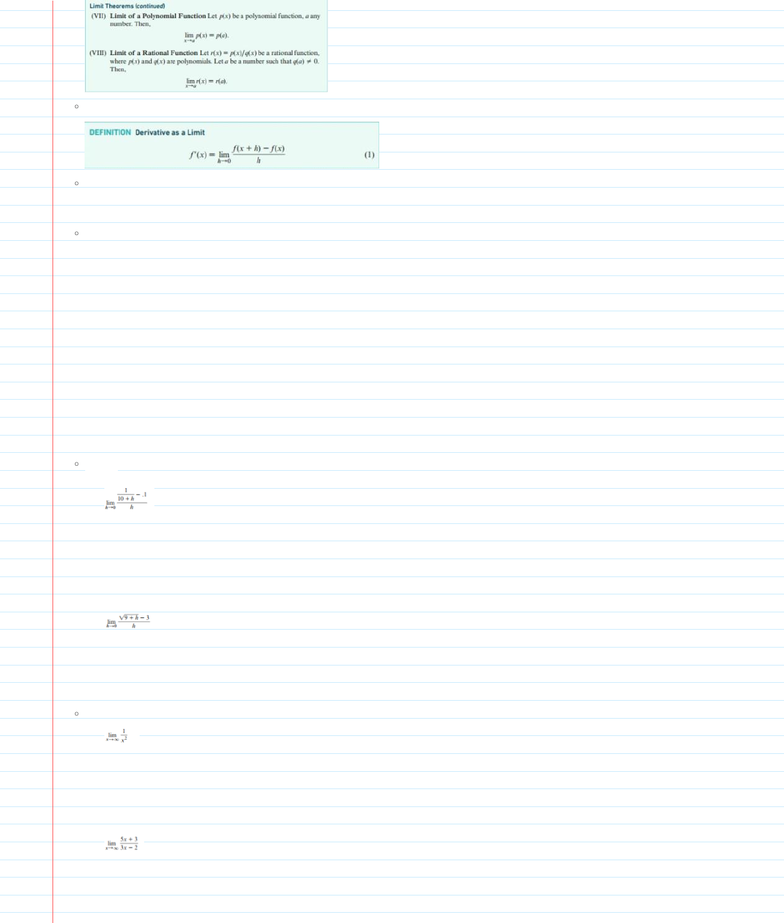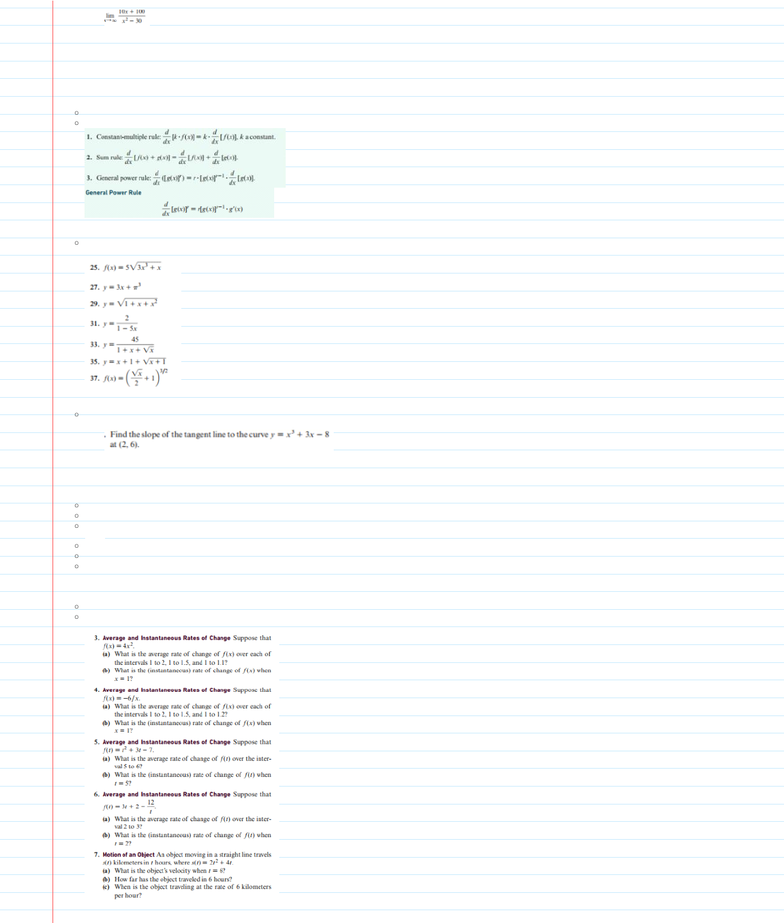# MATH221 Study Guide - Final Guide: Farad, Product Rule, Natural Logarithm

67 views36 pagesFind the slope at the following points using the equation y=x^2
Slope of a tangent line at point P= (change in y)/(change in x)
1.2: The Slope of a Curve at a Point
(-.4, .16)
a.
b.
(-1.5, 2.25)
c.
The derivative of f(x) is denoted by f'(x)
If f(x)=mx+b, then f'(x)=m
If f(x)=x^r, f(x)=r*x^r-1
The derivative is also the rate of change for an equation
To find the equation for the tangent line: y-f(a)=f'(a)(x-a)
The derivative is the slope of a line at a given point
The secant line is a line that passes through 2 points of f(x), whereas
the derivative only passes through 1 points
The slope of the secant line= [f(x+h)-f(x)] / [(x+h)-x]
f(x)=3x+7
1.
Find the derivative:
1.3: The Derivative
f(x)= (3x/4)-2
2.
f(x)=x^7
3.
f(x)=x^2/3
4.
f(x)= 1/(x)^5/2
5.
Lim g(x)=L
The limit of g(x) is L as it approaches a, if the values of g(x)
approach L as x approaches a
Limit Rules:
x--->a
1.4: Limits and the Derivative:
Final Exam Review
Monday, December 3, 2018
5:09 PM
FINAL EXAM REVIEW Page 1
Unlock document

This preview shows pages 1-3 of the document.
Unlock all 36 pages and 3 million more documents.Limit definition of the Derivative
Write the difference quotient (above)
1.
Simplify the difference quotient
2.
Find the limit as h-->0. This limit is f'(a)
3.
Calculate f'(a) using limits:
f(x)=3x+1
1.
Use limits to compute f'(x) for the given function:
f(x)=x+(1/x)
2.
f(x)=x/[x+1]
3.
1.
2.
Determine the function f(x) and the value of a for the following
equations
1.
2.
Compute the following limits:
3.
FINAL EXAM REVIEW Page 2
Unlock document

This preview shows pages 1-3 of the document.
Unlock all 36 pages and 3 million more documents.3.
1.6: Some Rules for Differentiation
Rules:
Differentiate:
1.
Solve:
1.8 The derivative as a Rate of Change
The rate of change of f(x) from a to b= [f(b)-f(a)] / [b-a]
The derivative f'(a) measures the instantaneous rate of change of f(x)
at x=a
s(t)= position
v(t)= velocity
s'(t)=v(t)
v'(t)=a(t)
s''(t)=a(t)
a(t)= acceleration
To approximate a change with the derivative: f(a+h)-f(a)~f'(a)*h
Practice:
FINAL EXAM REVIEW Page 3
Unlock document

This preview shows pages 1-3 of the document.
Unlock all 36 pages and 3 million more documents.

## Document Summary

1. 2: the slope of a curve at a point. Slope of a tangent line at point p= (change in y)/(change in x) Find the slope at the following points using the equation y=x^2 a. (-. 4, . 16) b. (-2, 4) c. (-1. 5, 2. 25) The derivative is the slope of a line at a given point. The derivative of f(x) is denoted by f"(x) The derivative is also the rate of change for an equation. To find the equation for the tangent line: y-f(a)=f"(a)(x-a) The secant line is a line that passes through 2 points of f(x), whereas the derivative only passes through 1 points. The slope of the secant line= [f(x+h)-f(x)] / [(x+h)-x] The limit of g(x) is l as it approaches a, if the values of g(x) approach l as x approaches a. Use limits to compute f"(x) for the given function: Determine the function f(x) and the value of a for the following equations.

## Get access